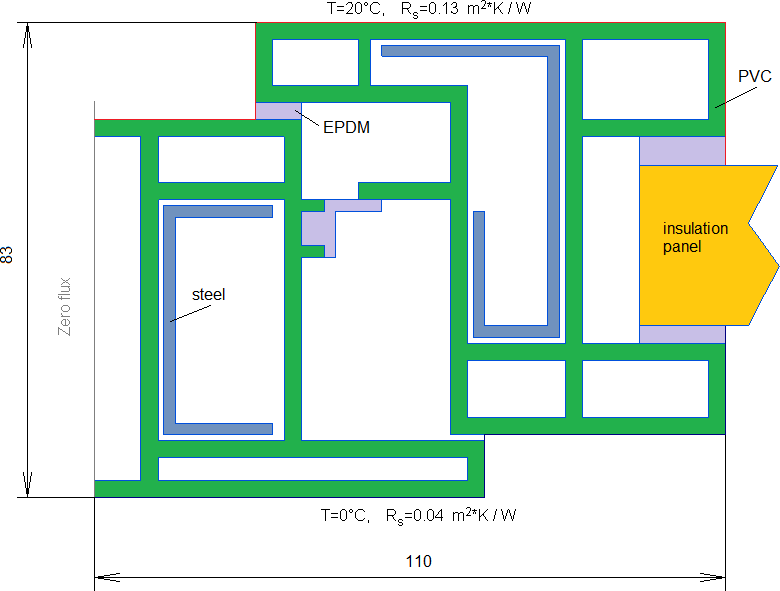QuickField

A new approach to field modelling
 Language: Global English Deutch Espanol Francais Italiano Danmark Ceske Chinese Pycckuü

Main >> Applications >> Sample problems >> Thermal transmittance in frames. Test case D.3 validation

# ISO 10077-2:2012 Test case D.3 - PVC frame section with steel reinforcement and insulation panel

QuickField simulation of the test case "D.3. PVC frame section with steel reinforcement and insulation panel" presented in ISO 10077-2:2012*

Problem Type:
Plane-parallel problem of heat transfer.

Geometry:total width b = 110 mm

Given:
Thermal conductivity of PVC, λ1 = 0.17 W/K·m.
Thermal conductivity of EPDM, λ2 = 0.25 W/K·m.
Thermal conductivity of insulation panel, λ3 = 0.035 W/K·m.
Thermal conductivity of steel λ4 = 50 W/K·m.

Problem:
Calculate two-dimensional thermal conductance L2D in accordance with ISO 10077-2:2012*.
(Reference thermal conductance L2D = 0.424 W/(m*K) )

Solution:
Air contact surface thermal resistance Rs is caused by convention mechanism of heat transfer (contrary to conductivity mechanism in case of two solid bodies in contact).
The convection coefficient value is reciprocal to the surface resistance value:
α20 = 1/0.13 W/(K·m2),
α0 = 1/0.04 W/(K·m2).

Thermal conductivities of air gaps are adjusted to include the convection and radiation heat transfers in real situation, and correlated with the actual temperatures on the gap contours according to the recommendation of ISO 10077-2:2012. Equivalent air gap conductivity calculations are automated in the accompanying Excel spreadsheet.

Results:
Heat flux and temperature distribution in frame section profile:Two-dimensional thermal conductance L2D = Heat flux per meter depth / Temperature difference = 8.58 / 20 = 0.429 W/(m*K).
Difference between the calculated and reference values is less than 3%. This simulation accuracy complies with the requirements of ISO 10077-2:2012.

• Video: